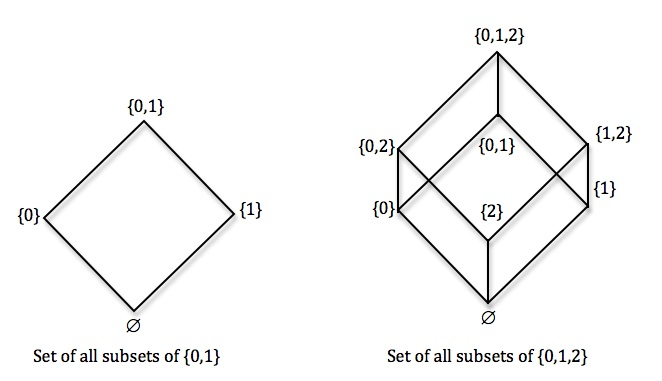# Astonishing consequences of naive thinking about sets: Cantor's diagonal argument

Posted by: Gary Ernest Davis on: October 4, 2010

I have mentioned in several posts now that many people do not think it is problematic to bundle all the counting numbers together in a set: the set of all natural numbers, denoted$\mathbb{N}$.

Another apparently harmless naive thought about set formation is that one can always construct the set of all subsets of a given set. For a set$A$ the set of all subsets of$A$ is denoted by$\mathcal{P}(A)$. For simple sets such as$A=\{ 0,1\} \textrm{ or } A=\{ 0,1,2\}$ we can easily visualize$\mathcal{P}(A)$ and the relations between its elements – i.e. the subsets of$A$:where$\emptyset$ denotes the empty set, and rising lines indicate the set below is a subset of the set above.

Perhaps someone might balk at reflecting on a set consisting of all subsets of the natural numbers, yet there is a simple device to make this construction seem plausible.

The set$\mathcal{P}(A)$ of all subsets of a set$A$ is in one-to-one correspondence with the set of all functions from the set$A$ into the two element set$\textbf{\underline{2}}:=\{ 0,1\}$.

This correspondence is established as follows: for each subset$B\subseteq A$ define a function$f:A \to \textbf{\underline{2}}$ by$f(x)=1 \textrm{ if } x\in B \textrm{ and } f(x)=0 \textrm{ if } x\notin B$; conversely, for each function$f:A\to \textbf{\underline{2}}$ define a subset$B\subseteq A$ of$A$ by$B=\{x\in A: f(x)=1\}$.

So to think about all subsets of the set$\mathbb{N}$ of natural numbers, it is equivalent, to think about all functions$f:\mathbb{N}\to \textbf{\underline{2}}$.

Such functions give a value of$0\textrm{ or } 1$ for each natural number, and so correspond to sequences$\epsilon_1,\epsilon_2,\epsilon_3,\ldots,$ where each$\epsilon_n$ is either$0 \textrm{ or } 1$.

Georg Cantor established the following extremely simple,  yet devastating, result: for any set$A$ for which the set$\mathcal{P}(A)$ of all subsets of$A$ exists, there cannot be a one-to-one correspondence between$A$ and$\mathcal{P}(A)$.

The argument is incredibly simple as it is elegant.

A one-to-one correspondence between$A$ and$\mathcal{P}(A)$ is established by a function$F:A \to \mathcal{P}(A)$ that is invertible.  Cantor shows that no function$F:A \to \mathcal{P}(A)$ is invertible. Here’s how he does it: let$F:A \to \mathcal{P}(A)$ be a function. Define the subset$B \textrm{ of } A$ by$B=\{ x \in A: x\notin F(x)\}$. Then, for every$x \in A, x \in F(x)$ exactly when$x \notin B$. Because either$x \in F(x) \textrm{ or } x \notin F(x)$ for every$x \in A$ it follows that$F(x) \neq B$ for all$x \in A$, and therefore the function$F$ is not invertible.

This is Cantor’s famous diagonal argument. Quite simple and relatively uncontroversial. Yet is has a devastating and astonishing consequence.

Given that, naively, we can always form the set of all subsets$\mathcal{P}(A)$ of a set$A$, we see that the set$\mathcal{P}(\mathbb{N})$ of all subsets of natural numbers cannot be put into one-to-one correspondence with$\mathbb{N}$ itself. Because the mapping$n \to \{n\}$ from$\mathbb{N} \textrm{ into } \mathcal{P}(\mathbb{N})$ is one-to-one, but not invertible, the set$\mathcal{P}(\mathbb{N})$ has greater cardinality than that of$\mathbb{N}$. That is, the set of all subsets of natural numbers is a set of greater order of infinity than the set of natural numbers itself.

What now stops us from forming the set$\mathcal{P}(\mathcal{P}(\mathbb{N}))$ which, by Cantor’s argument, has a higher order of infinity again, and so on, and so on(ad infinitum and beyond …) ? This is a glimpse of Cantor’s paradise from which, David Hilbert averred, no one shall expel us.

### 1 Response to "Astonishing consequences of naive thinking about sets: Cantor's diagonal argument"Great post.